# Introduction

Often the simulations from a model like APSIM will not be close enough to the observed data. APSIM (Classic and Next Generation) are very complex models with many moving pieces and unexpected interactions among parameters. If you decide that you need to optimize parameters I suggest answering these questions:

1. Why do you need to perform parameter optimization?

2. Which parameters do you need to optimize?

3. Are the observed measurements appropriate for the parameters you want to optimize?

I like to emphasize here the importance of thinking deeply about whether parameter optimization is needed in a given application. Before you use an optimization routine consider the following:

1. Did you control the quality of the weather data (‘met’ file)?

2. Are the soil properties appropriate for the location being simulated?

3. Is the management information accurate?

Clearly, if there are any problems, issues or inaccuracies which are derived from these three sources of information, then calibrating or tweaking crop parameters to compensate for those errors will not result in a useful model. In concrete terms, as an example: Are you modifying the radition use efficiency parameter to compensate for incorrect planting date or fertilizer inputs? Running an optimization algorithm is easier than having to think hard about the scientific questions, the mechanisms and the structure of the model.

Given this disclaimer, I provide simple functions which connect APSIM with optimization routines. These are optim_apsim and optim_apsimx. These functions work best for situations when the function being optimized is smooth (i.e. small changes in the inputs result in small changes in the output without jumps or discontinuities) and the parameters being optimized are continuous. This is: that both input parameters and outputs can take any real value. However, several of the optimization algorithms, such as the default ‘Nelder-Mead’ work for non-differentiable functions.

I have been testing them and they work as expected. This means that very often they give you the right answer to the wrong question! For example, the optimized value for a parameter might not be within a physical or biological meaningful range if you are not careful. Let’s look at the arguments:

## optim_apsim

• file = .apsim file
• src.dir = source directory. Should be the current directory for both this file and the crop file.
• crop.file = typically something like ‘Wheat.xml’, ‘Maize.xml’ or similar. You should have also placed an ‘ini’ under the crop in the .apsim file and point to this file.
• parm.paths = character vector with the full path to the parameters to be optimized. This can be obtained by using inspect_apsim or inspect_apsim_xml. The length of the parameter vector should be equal to the number of parameters being optimized (sometimes less is more). For example:
extd.dir <- system.file("extdata", package = "apsimx")
rue.pth <- inspect_apsim_xml("Maize75.xml", src.dir = extd.dir, parm = "rue")
## attrs:
## text: 0   0  1.60 1.60  1.60  1.60 1.60 1.40  1.30  1.30   0    0
## path: /Type/Model/rue
ext.pth <- inspect_apsim_xml("Maize75.xml", src.dir = extd.dir, parm = "y_extinct_coef")
## attrs: extinction coefficient for green leaf
## text: 0.70   0.55   0.55
## path: /Type/Model/y_extinct_coef
## To pass them to optim_apsim, combine them
pp <- c(rue.pth, ext.pth)

Generates the paths for the radiation use efficiency and extinction coefficient paths.

• data = data frame with the observed measurement which will be compared to APSIM output. It is important that the names match. This is the names given in the output of an APSIM simulation should have the same names as the column names in this data frame so that they can be aligned. Only one observation is allowed per Date.

• type = type of optimization. The default is to use optim. It is also possible to use the nloptr package or mcmc via the BayesianTools package (as of version 1.959).

• weights = weights to apply when multiple variables are being optimized. See help file for more details.

• index = the default is ‘Date’ this allows the alignment of APSIM output and observed measurements. If you have multiple simulations or ‘sites’ that you want to optimize, the index might need to be c(“outfile”, “Date”). In this way both the different simulations and observations for a particular ‘Date’ can be aligned. However, you need to construct the file with observed data carefully so that they can be aligned. (You need package version 1.975 or newer for this.)

• parm.vector.index = some paths point to a vector of parameters, but sometimes only one element of that vector needs to be optimized. In this case provide the index for which element of the vector needs to be optimized. If some of the parameters need this index while others do not, include a zero for those parameter vectors which are not indexed.

• … = additional arguments to be passed to optim. For example, you can include lower and upper bounds and change the optimization algorithm (in this case ‘L-BFGS-B’). Also, with ‘hessian = TRUE’ you can obtain confidence intervals. See optim help file and documentation. Other options for either nloptr or mcmc.

## optim_apsimx

This function has a very similar structure to optim_apsim. The main difference is that at the moment it is required to supply the initial values for the parameters. It is also required to indicate whether each parameter is present in the ‘replacement’ component. As expalined before, use inspect_apsimx or inspect_apsimx_replacement to find the parameter paths.

# Wheat Example

As a simple example, let’s imagine we have collected some data on phenology, LAI and biomass of wheat. The data are already in the package and we just need to load it. These data were actually simulated with known parameter values.

## Observed data

data(obsWheat)
## See the structure
head(obsWheat)
##           Date Wheat.Phenology.Stage Wheat.Leaf.LAI Wheat.AboveGround.Wt
## 275 2016-10-01              1.058553     0.00000000             0.000000
## 302 2016-10-28              3.483279     0.09736603             6.738461
## 329 2016-11-24              3.803049     0.63675398            33.700169
## 356 2016-12-21              3.804951     0.76458058            43.436635
## 383 2017-01-17              3.965591     0.73543599            51.434338
## 410 2017-02-13              4.837633     1.82638631            62.031093
## Only 10 observations
dim(obsWheat)
##  10  4
## Visualize the data
ggplot(obsWheat, aes(Date, Wheat.AboveGround.Wt)) +
geom_line() +
ggtitle("Biomass (g/m2)")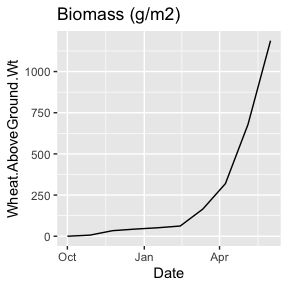ggplot(obsWheat, aes(Date, Wheat.Leaf.LAI)) +
geom_line() +
ggtitle("LAI")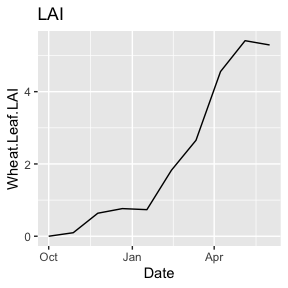ggplot(obsWheat, aes(Date, Wheat.Phenology.Stage)) +
geom_line() +
ggtitle("Phenology Stages")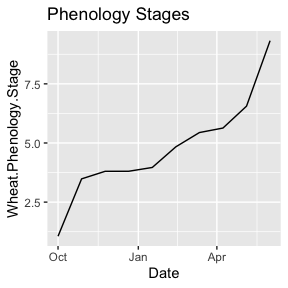Notice that the names in the obsWheat data frame are identical to the names from the APSIM-X output and this is important later in the optimization so that we can match the variables.

## Before optimization

sim0 <- apsimx("Wheat-opt-ex.apsimx", src.dir = extd.dir, value = "report")
## Select
sim0.s <- subset(sim0,
Date > as.Date("2016-09-30") & Date < as.Date("2017-07-01"))
## Visualize before optimization
## phenology
ggplot() +
geom_point(data = obsWheat, aes(x = Date, y = Wheat.Phenology.Stage)) +
geom_line(data = sim0.s, aes(x = Date, y = Wheat.Phenology.Stage)) +
ggtitle("Phenology")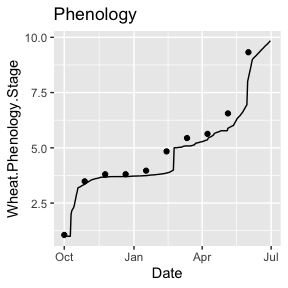## LAI
ggplot() +
geom_point(data = obsWheat, aes(x = Date, y = Wheat.Leaf.LAI)) +
geom_line(data = sim0.s, aes(x = Date, y = Wheat.Leaf.LAI)) +
ggtitle("LAI")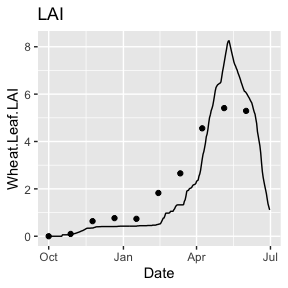## Biomass
ggplot() +
geom_point(data = obsWheat, aes(x = Date, y = Wheat.AboveGround.Wt)) +
geom_line(data = sim0.s, aes(x = Date, y = Wheat.AboveGround.Wt)) +
ggtitle("Biomass (g/m2)")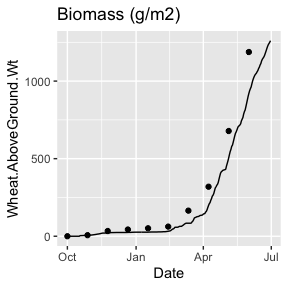## Setting up the optimization

We have observed data and we have ran the model. We notice that the agreement between observed and simulated could be better. For this example we will only be optimizing two parameters: RUE and phyllochron. We could use the function inspect_apsimx_replacement to find them. Then we construct the paths.

## Finding RUE
inspect_apsimx_replacement("Wheat-opt-ex.apsimx", src.dir = extd.dir,
node = "Wheat",
node.child = "Leaf",
node.subchild = "Photosynthesis",
node.subsubchild = "RUE",
parm = "FixedValue",
verbose = FALSE)
## $type : Models.Functions.Constant, Models ## FixedValue : 1.2 ## Finding BasePhyllochron inspect_apsimx_replacement("Wheat-opt-ex.apsimx", src.dir = extd.dir, node = "Wheat", node.child = "Cultivars", node.subchild = "USA", node.subsubchild = "Yecora", verbose = FALSE) ##$type : Models.PMF.Cultivar, Models
##  : [Phenology].MinimumLeafNumber.FixedValue = 6
##  : [Phenology].VrnSensitivity.FixedValue = 0
##  : [Phenology].PpSensitivity.FixedValue = 4
##  : [Structure].Phyllochron.BasePhyllochron.FixedValue  = 120
##  : [Leaf].CohortParameters.MaxArea.AreaLargestLeaves.FixedValue = 4000
## Command
## Name : Yecora
## IncludeInDocumentation : TRUE
## Enabled : TRUE
## ReadOnly : FALSE
## Constructing the paths is straight-forward
pp1 <- "Wheat.Leaf.Photosynthesis.RUE.FixedValue"
pp2 <- "Wheat.Cultivars.USA.Yecora.BasePhyllochron"

## Running the optimization

Once we have set up these pieces we can run the optimization. Here, the first argument is the name of the file, the second the directory where it is (src.dir), then the paths indicating the parameters to optimize, the observed data (data), the weighting method (here = mean), whether the parameters are in the replacement part of the simulation and the initial values.

## wop is for wheat optimization
wop <- optim_apsimx("Wheat-opt-ex.apsimx",
src.dir = extd.dir,
parm.paths = c(pp1, pp2),
data = obsWheat,
weights = "mean",
replacement = c(TRUE, TRUE),
initial.values = c(1.2, 120))

This optimization ran for about 7 minutes (on my laptop). This is the result:

wop
## Initial values:
##   Parameter path:  Wheat.Leaf.Photosynthesis.RUE.FixedValue
##   Values:  1.2
##   Parameter path:  Wheat.Cultivars.USA.Yecora.BasePhyllochron
##   Values:  120
## Optimized values:
##   Parameter path:  Wheat.Leaf.Photosynthesis.RUE.FixedValue
##   Values:  1.494
##   Parameter path:  Wheat.Cultivars.USA.Yecora.BasePhyllochron
##   Values:  87.631
## Convergence: 0

We can see that the optimized values are very close to the known values which were used to simulate the data. The known value for RUE was 1.5 $$g \; MJ^{-1}$$ and for BasePhyllochron it was 90 GDD.

In addition, it is good practice to compute the Hessian matrix, since it provides information about standard errors and confidence intervals.

## wop is for wheat optimization
wop.h <- optim_apsimx("Wheat-opt-ex.apsimx",
src.dir = extd.dir,
parm.paths = c(pp1, pp2),
data = obsWheat,
weights = "mean",
replacement = c(TRUE, TRUE),
initial.values = c(1.2, 120),
hessian = TRUE)

This ran for about 8 minutes and it allows for calculation of confidence intervals and standard errors.

wop.h
## Initial values:
##   Parameter path:  Wheat.Leaf.Photosynthesis.RUE.FixedValue
##   Values:  1.2
##   Parameter path:  Wheat.Cultivars.USA.Yecora.BasePhyllochron
##   Values:  120
## Optimized values:
##   Parameter path:  Wheat.Leaf.Photosynthesis.RUE.FixedValue
##   Values:  1.494
##   CI level:  0.95 t:  2.306004  SE: 0.003
##   Lower:  1.487   Upper:  1.502
##   Parameter path:  Wheat.Cultivars.USA.Yecora.BasePhyllochron
##   Values:  87.631
##   CI level:  0.95 t:  2.306004  SE: 0.29
##   Lower:  86.962  Upper:  88.299
## Convergence: 0

After optimization there is good agreement between observed and simulated.

## We re-run the model because the Wheat-opt-ex.apsimx file
sim.opt <- apsimx("Wheat-opt-ex.apsimx", src.dir = extd.dir, value = "report")
sim.opt.s <- subset(sim.opt,
Date > as.Date("2016-09-30") & Date < as.Date("2017-07-01"))
  ## phenology
ggplot() +
geom_point(data = obsWheat, aes(x = Date, y = Wheat.Phenology.Stage)) +
geom_line(data = sim.opt.s, aes(x = Date, y = Wheat.Phenology.Stage)) +
ggtitle("Phenology")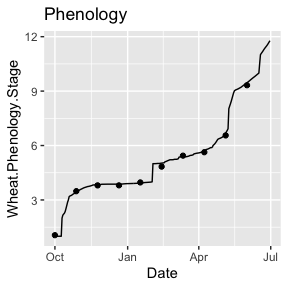## LAI
ggplot() +
geom_point(data = obsWheat, aes(x = Date, y = Wheat.Leaf.LAI)) +
geom_line(data = sim.opt.s, aes(x = Date, y = Wheat.Leaf.LAI)) +
ggtitle("LAI")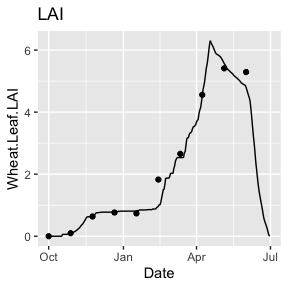## Biomass
ggplot() +
geom_point(data = obsWheat, aes(x = Date, y = Wheat.AboveGround.Wt)) +
geom_line(data = sim.opt.s, aes(x = Date, y = Wheat.AboveGround.Wt)) +
ggtitle("Biomass (g/m2)")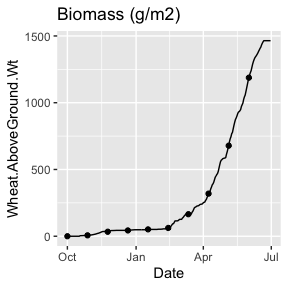## Multi-simulation optimization

In both APSIM Classic and Next Generation it might be common to perform simulations (for example for different sites or years). In this case the optimization might be done over those multiple simulations. The main considerations when doing this is that for Classic the observations should be organized with ‘outfile’ for the first column. This column there whould be a string which matches the simulation in APSIM that corresponds to this specific simulation (i.e. site/year). The second column should be the ‘Date’ and the variables to be optimized should match those names in the APSIM simulation. Also, the argument ‘index’ in ‘optim_apsim’ should be c(‘outfile’, ‘Date’). For Next Generation, the considerations are very similar except that the observed data should be organized with ‘report’ as the first column and ‘Date’ as the second column. Likewise, the ‘index’ argument in ‘optim_apsimx’ should be c(‘report’, ‘Date’). In this way, for both types of optimizations the simulations in APSIM will be able to be matched with the observations.

### Additional considerations and potential issues

The main additional consideration is that it can be a good idea to set up lower and upper bounds on the parameters in terms of how much higher and lower than the default values can be. In a model such as APSIM usually plus or minus 50 percent is enough of a range and even a lower range can often suffice. These can be included as 0.5 and 1.5. With optim it is necessary to use method = “L-BFGS-B”. There are also similar options when using nloptr. This package has many available algorithms to choose from with more modern implementations than the ones available in optim. However, one disadvantage of the nloptr function is that it does not seem to be able to compute the Hessian numerically.

• One issue which I have not fully tracked down is an error which appears which indicates a conflict with disk I/O. Again, I need to check but this may be when trying to read and/or write an apsimx file which is also opened in the APSIM GUI application. It is possible that this issue goes away when the APSIM GUI is closed.

• I’m not convinced that using the Hessian (in the way I’m doing it) produces accurate standard errors. It seems to work just fine when there is one variable and perhaps when there is no weighting applied, but it seems that it breaks down with multiple variables and weighting. The mcmc method should not have this problem, but it takes a long time to run.